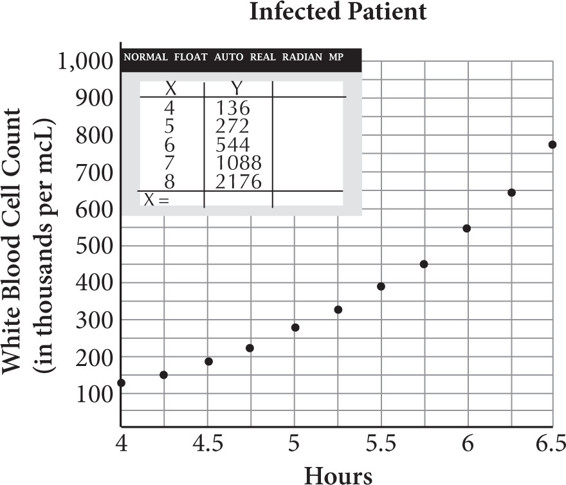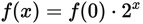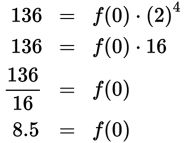# SAT Math Multiple Choice Question 386: Answer and Explanation

### Test Information

Question: 386

11.Typically, when people contract an infectious disease, their immune system immediately begins to produce extra white blood cells to fight the disease. The scatterplot shows the white blood cells reproducing in an infected patient, along with several values found when modeling the data using a graphing calculator. According to this model, how many white blood cells per microliter of blood did the patient have before he contracted the disease?

• A. 3,400
• B. 8,500
• C. 10,000
• D. 13,600

Explanation:

B

Difficulty: Medium

Category: Problem Solving and Data Analysis / Scatterplots

Strategic Advice: Translate from English into math: The number of cells in the original sample means the value of the function at 0 hours, or f(0). The first x-value on the graph is 4, not 0, so you'll need to use the values shown in the calculator screenshot to write an equation for the model.

Getting to the Answer: Notice that the y-values in the calculator screenshot double as the x-values increase by 1. This means that the model is an exponential growth function of the form. Choose a point from the calculator screenshot such as (4, 136), substitute the values into the function, and solve for f(0):The y-axis title tells you that the numbers are given in thousands, so there were 8,500 white blood cells per microliter in the original sample.

You could also work backward from the calculator screenshot by dividing by 2 four times (from 4 hours to 3 hours, 3 hours to 2 hours, 2 hours to 1 hour, and 1 hour to before the patient contracted the disease). The result is 136 ÷ 24 = 136 ÷ 16 = 8.5, which in thousands is 8,500.